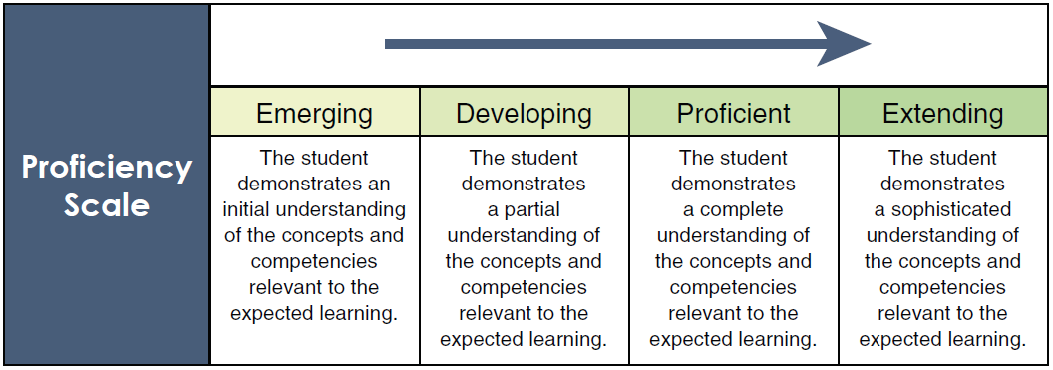## Mathematics 8

Mathematics 8 Course Overview

Big Ideas

 Numbers represent quantities that canbe decomposed into smaller parts. One-to-one correspondence and a sense of 5 and 10are essential for fluency with numbers. Repeating elements in patterns can be identified. Objects haveattributes that can be described, measured, and compared. Familiar eventscan be describedas likely or unlikelyand compared.

Introduction

You will continue to develop your math competencies in Reasoning & Analyzing, Understanding & Solving, Communicating & Representing and Connecting & Reflecting while extending and broadening knowledge of topics that you have learned during your elementary years.

You will extend your knowledge to other types of numbers such as square roots and fractions, apply what you know about ratios to deal with the concept of rate and proportions, solve more complex equations, deal with graphs that involve larger numbers and find surface area and volume of 3-D objects.

Where does this course fit?

• Pre-requisite: Math 7
• Required to continue to Math 9

Course Materials

• Course materials provided online
• Scientific calculator allowed on all course tasks
• Some graphing paper needed
• Math Makes Sense 8 textbook available to borrow

Brief Outline

 Unit Description Percents You will learn how to deal with percentages less than 1 and greater than 100 Squares, Cubes and Pythagoras You will learn how to calculate the square or cube root of a number and how to use the Pythagorean theorem to solve problems Rates and Proportions You will extend your knowledge of ratios to solve problems involving numerical proportional reasoning Fractions You will learn how to add, subtract, multiply and divide different types of fractions Linear Equations You will learn how to solve multi-step linear equations Geometry & Nets You will learn how to visualize different views of 3D objects Surface Area & Volume You will learn how to use areas of 2D figures to calculate surface area and volume of 3D objects Probability You will learn about different ways to look at a set of data and solve theoretical probability for different situations Financial Literacy You will apply what you know about rates and percentages to find the best deal

Assessment Percentage Breakdown

 Assessment Type Percentage of the Course Assignments 20% Quizzes 20% Projects 10% Midterm + Final Exam 20% + 30%

Your final assessment will be represented on this Proficiency Scale.You have up to a year to complete your course.